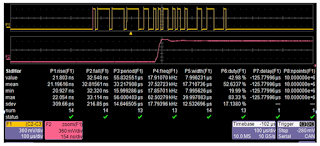## You need to test, we're here to help.

You need to test, we're here to help.

## 06 December 2017

### Probing Techniques and Tradeoffs (Part IV)Figure 1: Applying bandwidth filters to a 2.5-GHz clock signal clearly shows the effect of bandwidth on rise time
The topic of probe bandwidth is a broad and deep one. We began our discussion of bandwidth in an earlier post with some basic information about what bandwidth means and the importance of the -3 dB point. Next, we looked at a Fourier deconstruction of a square wave into its fundamental and the lower-order harmonics, and covered the importance of bandwidth in capturing enough harmonic content to understand the signal's overall shape.

Now we'll move on to examine the relationship between bandwidth and the rise time of your signal. Figure 1 shows a capture of a 2.5-GHz clock signal made with a 20-GHz oscilloscope, which allows us to capture the fundamental frequency and beyond, out to the seventh harmonic. If we apply various bandwidth filters, we can see how the waveshape changes. For example, the third trace from the top at left in Figure 1 shows the acquisition with a 13-GHz filter, with which we are acquiring about 50% of the power contained in the fifth harmonic. The measured rise time is about 82 ps.

If we apply even more filtering to the oscilloscope's front end, we can see the wave shape being reduced to the fundamental component. The second trace from the top at right in Figure 1 shows a 4-GHz filter applied to the acquisition. In this case, the rise time is about 106 ps. These images give you an idea of how bandwidth limiting affects the shape of a captured signal.Figure 2: A 125-kb/s CAN signal has a low bit rate but a very fast rise time of 21 ns
It's important to note the fastest rise time of your signal to ensurer that you have enough bandwidth available for accurate characterization. Signals that seem to be low in speed can still have high-frequency content that requires a high sample rate.

In particular, digital signals can have a relatively low bit rate but a very fast rise time. Figure 2 shows an acquisition of a 125-kb/s CANbus signal. The unit interval of this signal is 8 μs, but its rise time of 21 ns is 300X faster than the UI. The signal contains frequency content up to 21.5 MHz. The analog bandwidth required to characterize a signal is derived from this expression:

Bandwidth = 0.45/rise timeFigure 3:
One must also consider the bandwidth of the oscilloscope-plus-probe system, as the cascading of elements without compensation can reduce the total system bandwidth. For example, if we were to connect a 20-GHz probe to a 20-GHz oscilloscope, with each element having a -3 dB bandwidth of 20 GHz, we would end up with a total system bandwidth of only 14 GHz (Figure 3).

Obviously, this is far from an ideal situation. We expect the cascaded system to operate out to 20 GHz. The means of achieving this is to use system frequency-response compensation, which is applied to correct for the attenuation we would observe by cascading the 20-GHz probes and 20-GHz oscilloscope.Figure 4: These graphs illustrate the effects of frequency-response compensation on total system bandwidth
Some of this frequency-response compensation is applied in the design of the elements themselves, some in the matching networks of the differential amplifier, and some in calibration when the probes are manufactured. Additionally, the frequency response of the probes is characterized and compensated for in software. When all is said and done, the combination of the 20-GHz probes and 20-GHz oscilloscope delivers a total system bandwidth of 20 GHz with compensated attenuation of 2 dB as opposed to 6 dB without compensation (Figure 4).

Our next installment will take up the topic of probe loading in more detail. Meanwhile, please visit the prior entries in this series of posts:

Probing Techniques and Tradeoffs (Part I)
Probing Techniques and Tradeoffs (Part II)
Probing Techniques and Tradeoffs (Part III)